# Binomial Theorem and Mathematical Induction Notes Class 11th Maths

0
1436

Binomial Theorem for Positive Integer

If n is any positive integer, then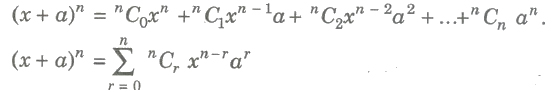This is called binomial theorem.

Here, nC0nC1nC2, … , nno are called binomial coefficients and

nCr = n! / r!(n – r)! for 0 ≤ r ≤ n.

Properties of Binomial Theorem for Positive Integer

(i) Total number of terms in the expansion of (x + a)n is (n + 1).

(ii) The sum of the indices of x and a in each term is n.

(iii) The above expansion is also true when x and a are complex numbers.

(iv) The coefficient of terms equidistant from the beginning and the end are equal. These coefficients are known as the binomial coefficients and

nCr = nCn – r, r = 0,1,2,…,n.

(v) General term in the expansion of (x + c)n is given by

Tr + 1 = nCrxn – r ar.

(vi) The values of the binomial coefficients steadily increase to maximum and then steadily decrease .

(vii)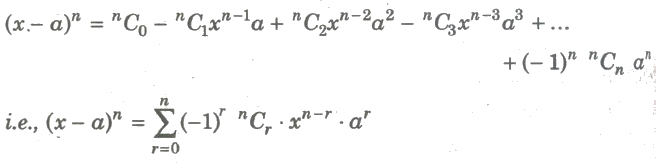(viii)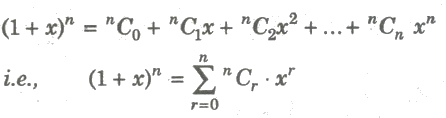(ix) The coefficient of xr in the expansion of (1+ x)n is nCr.

(x)

(xi) (a)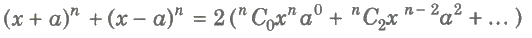(b)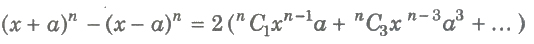(xii) (a) If n is odd, then (x + a)n + (x – a)n and (x + a)n – (x – a)n both have the same number of terms equal to (n +1 / 2).

(b) If n is even, then (x + a)n + (x – a)n has (n +1 / 2) terms. and (x + a)n – (x – a)n has (n / 2) terms.

(xiii) In the binomial expansion of (x + a)n, the r th term from the end is (n – r + 2)th term from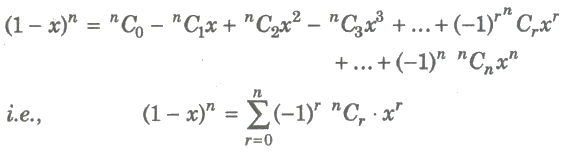the beginning.

(xiv) If n is a positive integer, then number of terms in (x + y + z)n is (n + l)(n + 2) / 2.

Middle term in the Expansion of (1 + x)n

(i) It n is even, then in the expansion of (x + a)n, the middle term is (n/2 + 1)th terms.

(ii) If n is odd, then in the expansion of (x + a)n, the middle terms are (n + 1) / 2 th term and (n + 3) / 2 th term.

Greatest Coefficient

(i) If n is even, then in (x + a)n, the greatest coefficient is nCn / 2

(ii) Ifn is odd, then in (x + a)n, the greatest coefficient is nCn – 1 / 2 or nCn + 1 / 2 both being equal.

Greatest Term

In the expansion of (x + a)n

(i) If n + 1 / x/a + 1 is an integer = p (say), then greatest term is Tp == Tp + 1.

(ii) If n + 1 / x/a + 1 is not an integer with m as integral part of n + 1 / x/a + 1, then Tm + 1. is the greatest term.

Important Results on Binomial Coefficients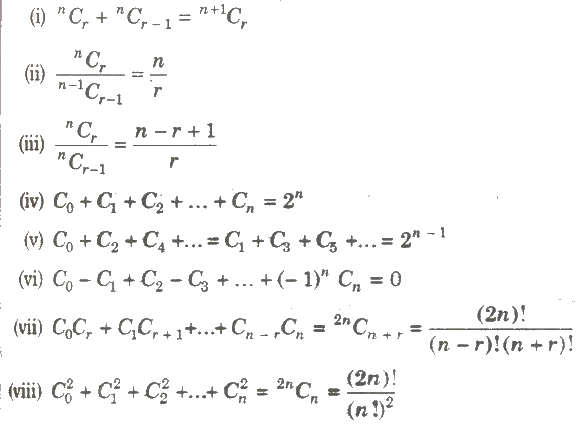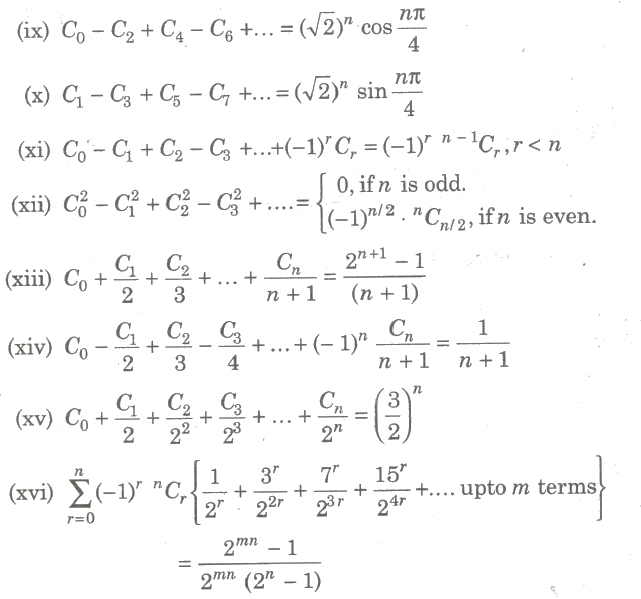Divisibility Problems

From the expansion, (1+ x)n = 1+ nC1x + nC1x2+ … +nCnxn

We can conclude that,

(i) (1+ x)n – 1 = nC1x + nC1x2+ … +nCnxn is divisible by x i.e., it is multiple of x.

(1+ x)n – 1 = M(x)

(ii)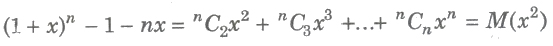(iii)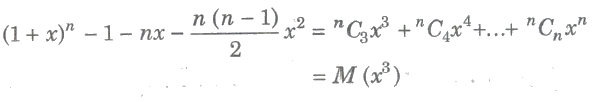Multinomial theorem

For any n ∈ N,

(i)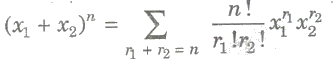(ii)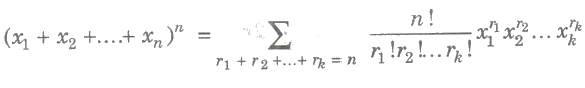(iii) The general term in the above expansion is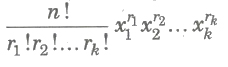(iv)The greatest coefficient in the expansion of (x1 + x2 + … + xm)n is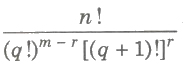where q and r are the quotient and remainder respectively, when n is divided by m.

(v) Number of non-negative integral solutions of x1 + x2 + … + xn = n is n + r – 1Cr – 1

R-f Factor Relations

Here, we are going to discuss problem involving (√A + B)sup>n = I + f, Where I and n are positive integers.

0 le; f le; 1, |A – B2| = k and |√A – B| < 1

Binomial Theorem for any Index

If n is any rational number, then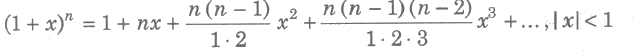(i) If in the above expansion, n is any positive integer, then the series in RHS is finite otherwise infinite.

(ii) General term in the expansion of (1 + x)n is Tr + 1 = n(n – 1)(n – 2)… [n – (r – 1)] / r! * xr

(iii) Expansion of (x + a)n for any rational index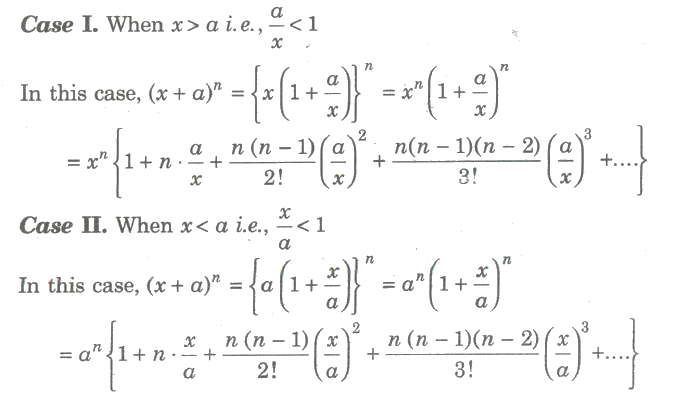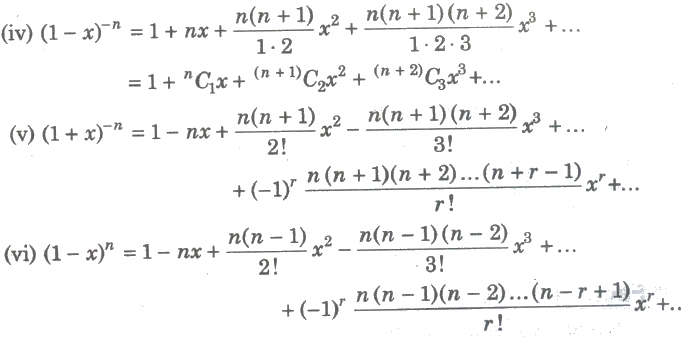(vii) (1 + x)– 1 = 1 – x + x2 – x3 + …∞

(viii) (1 – x)– 1 = 1 + x + x2 + x3 + …∞

(ix) (1 + x)– 2 = 1 – 2x + 3x2 – 4x3 + …∞

(x) (1 – x)– 2 = 1 + 2x + 3x2 – 4x3 + …∞

(xi) (1 + x)– 3 = 1 – 3x + 6x2 – …∞

(xii) (1 – x)– 3 = 1 + 3x + 6x2 – …∞

(xiii) (1 + x)n = 1 + nx, if x2, x3,… are all very small as compared to x.

Important Results

(i) Coefficient of xm in the expansion of (axp + b / xq)n is the coefficient of Tr + l where r = np – m / p + q

(ii) The term independent of x in the expansion of axp + b / xq)n is the coefficient of Tr + lwhere r = np / p + q

(iii) If the coefficient of rth, (r + l)th and (r + 2)th term of (1 + x)n are in AP, then n2 – (4r+1) n + 4r2 = 2

(iv) In the expansion of (x + a)n

Tr + 1 / Tr = n – r + 1 / r * a / x

(v) (a) The coefficient of xn – 1 in the expansion of

(x – l)(x – 2) ….(x – n) = – n (n + l) / 2

(b) The coefficient of xn – 1 in the expansion of

(x + l)(x + 2) ….(x + n) = n (n + l) / 2

(vi) If the coefficient of pth and qth terms in the expansion of (1 + x)n are equal, then p + q = n + 2

(vii) If the coefficients of xr and xr + 1 in the expansion of a + x / b)n are equal, then

n = (r + 1)(ab + 1) – 1

(viii) The number of term in the expansion of (x1 + x2 + … + xr)n is n + r – 1C r – 1.

(ix) If n is a positive integer and a1, a2, … , am ∈ C, then the coefficient of xr in the expansion of (a1 + a2x + a3x2 +… + amxm – 1)n is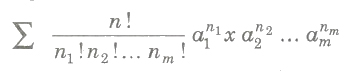(x) For |x| < 1,

(a) 1 + x + x2 + x3+ … + ∞ = 1 / 1 – x

(b) 1 + 2x + 3x2 + … + ∞ = 1 / (1 – x)2

(xi) Total number of terms in the expansion of (a + b + c + d)n is (n + l)(n + 2)(n + 3) / 6.

Important Points to be Remembered

(i) If n is a positive integer, then (1 + x)n contains (n +1) terms i.e., a finite number of terms. When n is general exponent, then the expansion of (1 + x)n contains infinitely many terms.

(ii) When n is a positive integer, the expansion of (l + x)n is valid for all values of x. If n is general exponent, the expansion of (i + x)n is valid for the values of x satisfying the condition |x| < 1.

Previous articlePermutations & Combinations Notes Class 11th Maths